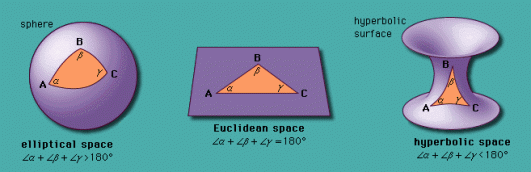# What Are The Kinds Of Geometry?

Geometry has three basic types or "kinds" in terms of mathematics. An easier way to describe the difference between these three types is to compare each geometrys definition of a triangle.

These three types are:• Hyperbolic Geometry - In which the sum totals of angles of a triangle must add up to less than 180 degrees this is non-Euclidian as it's not viewed in a flat space.
• Euclidian Geometry - Traditional geometry which is viewed on a infinite flat plane in 2D. The principle here is that triangles should have 3 angles which equate to a total of 180 degrees.
• Elliptic Geometry  -  In Elliptic Geometry triangles should have three angles which total higher than 180 degrees.
To understand the difference between these three types of geometry  we must first understand the meaning of geometry in mathematical terms.

Geometry in Maths

Now I can't profess to be anything of a maths genius but I did find this video which gives a good grounding in the basic principles of geometry!

thanked the writer.